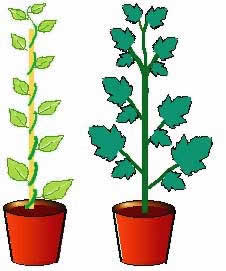#### You may also like### I'm Eight

Find a great variety of ways of asking questions which make 8.### Let's Investigate Triangles

Vincent and Tara are making triangles with the class construction set. They have a pile of strips of different lengths. How many different triangles can they make?### Noah

Noah saw 12 legs walk by into the Ark. How many creatures did he see?

# The Tomato and the Bean

## The Tomato and the Bean

Tom's Dad sowed some tomato seed in February. He gave Tom one of the tomato plants in a pot.

At the beginning of May Tom put his tomato plant outside. On the same day he sowed a bean in another pot.

Ten days later the bean plant was just $1$ cm (centimetre) above the soil surface. Tom measured his tomato plant which was already $38$ cm tall.

Each evening Tom measured his two plants.

On the evening of the next day the little bean plant had grown another $2$ cm so it was $3$ cm high. Each day it continued to grow double the amount it had grown the day before.

The tomato plant grew at a steady $5$ cm a day.After how many days were the two plants the same height when Tom measured them in the evening? How high were they?

### Why do this problem?

This problem can help to give learners an understanding of the difference between a steady and an increasing rate of growth. It could be used when looking at number patterns, especially doubling. It puts patterns in a real context, and can be used to demonstrate the use of tables in problem solving. It will also be fascinating for you, as the teacher, to see how children represent the problem while they work on it.

### Possible approach

You could start this problem as a whole class activity by introducing the growth rate of other plants as examples. (These plants could be real or imagined!) For example:
"There is a plant that is $3$ centimetres tall. If it grows $4$ centimetres a day how tall will it be the next day?" "And the day after that?" And so on ...

Then you can introduce a different type of example:
"There is a plant that is $5$ centimetres tall. If it doubles in height each day how tall will it be the next day?""And the day after that?" And so on ...

Then a new question needs to be asked to help in approaching the problem as written. For example:
"It starts at $5$ centimetres and is $10$ centimetres the next day. How much has it grown?"
"If it grows double that the next day, how tall will it be?"

You could then ask learners to do the given problem in pairs and encourage them to use anything they like to help them with the solution. Some may draw pictures and use numbers, some may make a list, some may use multilink to physically represent the plants, some will be able to make a table of the results, others may be happier if given this sheeet with a table to be completed.

### Key questions

At what height did the bean start?
How tall was it the next day?
How much did it grow by the next day? So how tall would it be that day?
And the day after that?
At what height did the tomato start?
How tall would it be on the second day? And the day after that?

### Possible extensions

Learners can be encouraged to open out this activity further by considering plants that increase their rate of growing by trebling/quadrupling the growth each year. Each year's height could be calculated and learners can see what happens.
Doubling goes:- $1$, $3$, $7$, $15$, $31$, $63$ ...
Trebling goes:- $1$, $4$, $13$, $40$ ...
Quadrupling goes, $1$, $5$, $21$, $85$ ...

To open this problem out further, children may like to explore the digital roots of the numbers in their results. This sheet gives some suggestions as to how this might be done.

### Possible support

Suggest using this sheet and filling in first the days ($1$-$8$) and the heights on the first day. The bean's growth is easier to work out than the tomato's so this can be tackled next.